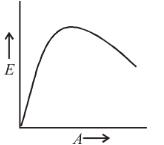# Nuclear Physics MCQ Level – 1

## 10 Questions MCQ Test Modern Physics for IIT JAM | Nuclear Physics MCQ Level – 1

Description
This mock test of Nuclear Physics MCQ Level – 1 for Physics helps you for every Physics entrance exam. This contains 10 Multiple Choice Questions for Physics Nuclear Physics MCQ Level – 1 (mcq) to study with solutions a complete question bank. The solved questions answers in this Nuclear Physics MCQ Level – 1 quiz give you a good mix of easy questions and tough questions. Physics students definitely take this Nuclear Physics MCQ Level – 1 exercise for a better result in the exam. You can find other Nuclear Physics MCQ Level – 1 extra questions, long questions & short questions for Physics on EduRev as well by searching above.
QUESTION: 1

### Order of ionization power of α,β, and γ rays is

Solution:

Since α rays has more charge than β and γ has no charge therefore order of ionization is α > β > γ.

The correct answer is: α > β > γ

QUESTION: 2

### The binding energies of the nuclei Pn and  Q2n are x and y  joules. If 2x > y  then the energy released in the reaction  Pn + Pn → Q2n,  will be

Solution:

The correct answer is: -(2x - y)

QUESTION: 3

### A radioactive element Th(84Po216) can undergo α and β are type of disintegration with half lives, T1 and T2 respectively. Then the half life of Th is .

Solution: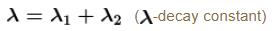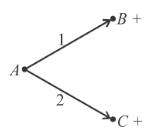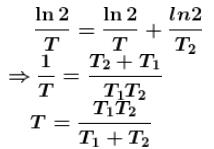The correct answer is: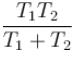QUESTION: 4

The order of magnitude of the density of nuclear matter is :

Solution:

The correct answer is: 1017 kg/m

QUESTION: 5

The value of binding energy for 1H22He428Fe5692U235  are 2.22, 28.3, 492 and 1786 units respectively. The most stable nucleus is :

Solution:

QUESTION: 6

The specific activity of radium is nearly

Solution:

QUESTION: 7

In equation 92U235 + 0n→ 56Ba144 + 36Kr89 + X ; X is :

Solution:

The correct answer is: 3 0n1

Nuclear fission equation

92U235 +  0n→ 56Ba144 + 36Kr89 + 3 0n
Hence particle x is neutron.

QUESTION: 8

Masses of nucleus, neutron an protons are M, mn and mrespectively. If nucleus has been divided into neutrons and protons, then

Solution:

The correct answer is: M < (A - Z)mn + Zmp

QUESTION: 9

In most stable nuclei neutron number N and proton number Z has the relation

Solution:

The correct answer is: N ≥ Z

QUESTION: 10

The graph between the binding energy per nucleon (E) and atomic mass number (A) is as :

Solution:

The correct answer is: